# RD Sharma Solutions For Class 7 Maths Chapter - 9 Ratio And Proportion

RD Sharma Solutions for Class 7 Maths Chapter 9 Ratio and Proportion is the best study material for students who are wishing to secure excellence in board exams. The PDF of this chapter are available here, and students can download for free. RD Sharma Solutions are useful for students as it helps them in scoring high marks in the examination. These solutions are formulated by subject matter experts of BYJU’S with utmost care.

Chapter 9, Ratio and Proportion, has three exercises. RD Sharma Solutions for Class 7 given here contains the solutions to all the questions present in these exercises. Let us have a look at some of the concepts that are being discussed in this chapter.

• Ratio – the ratio of two quantities of the same kind and in the same units is a fraction that shows how many times the one quantity is of the other
• Ratio in simplest form
• Comparison of ratios
• Definition and meaning of equivalent ratios
• Proportion – equality of two ratios is called proportion
• Definition of mean proportional

## Download the PDF of RD Sharma Solutions For Class 7 Maths Chapter 9 Ratio and Proportion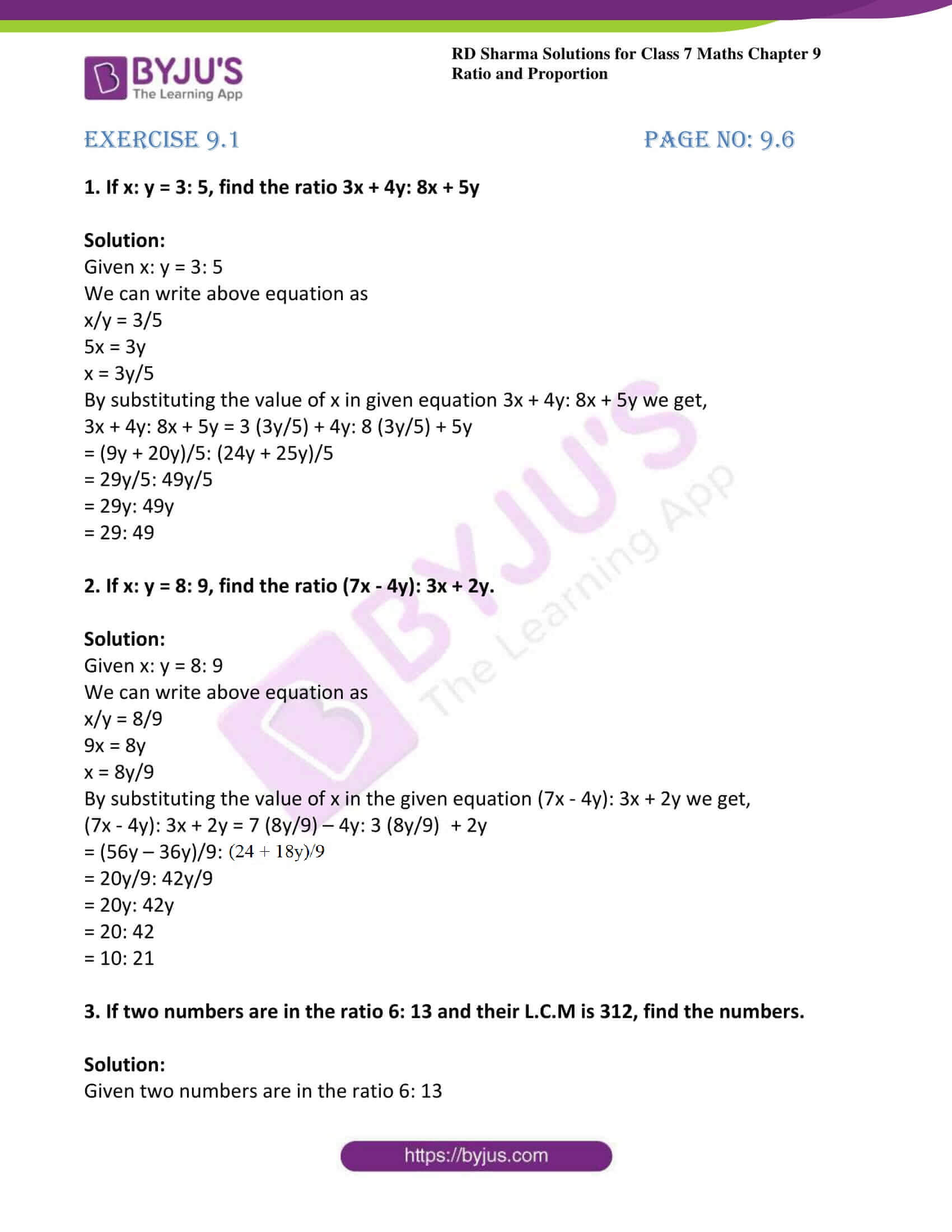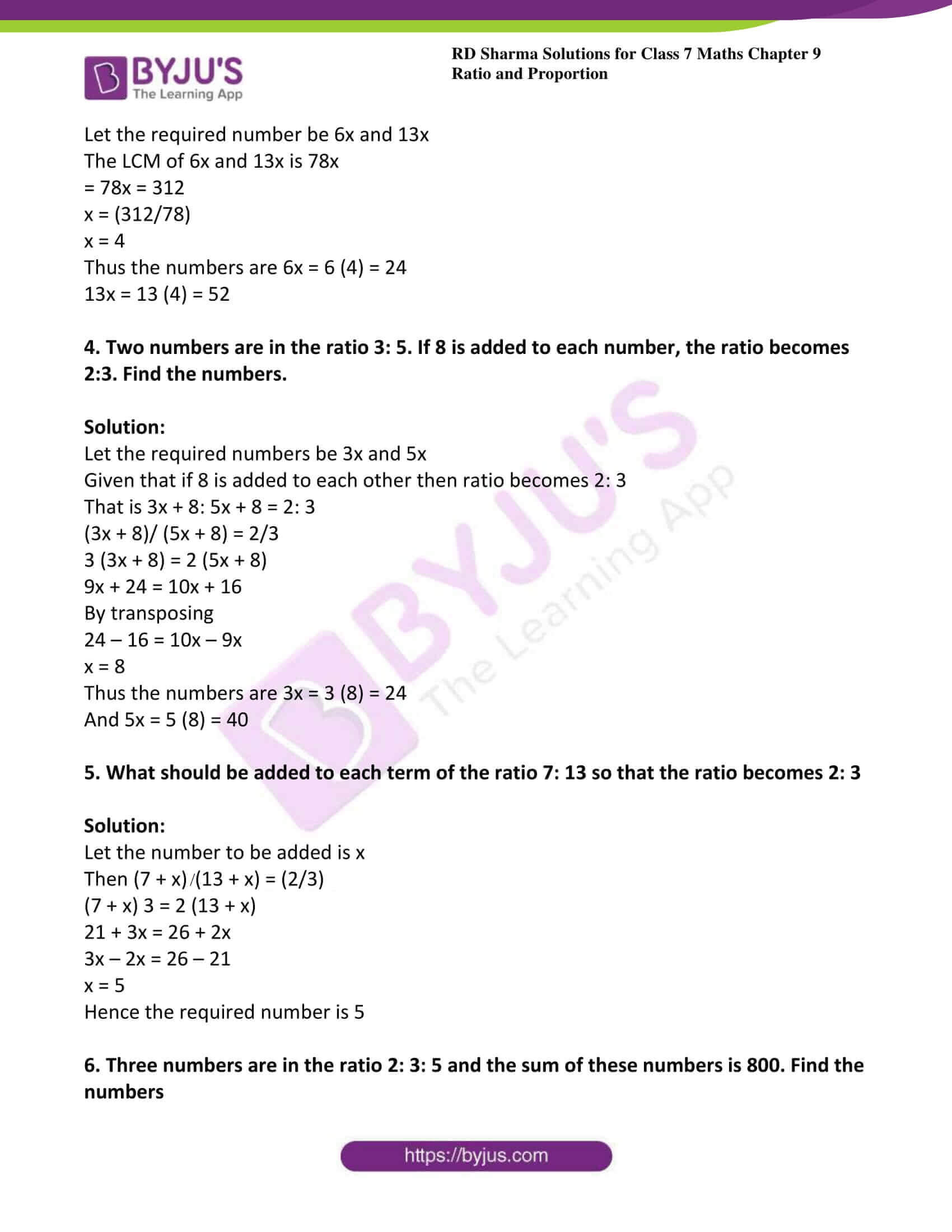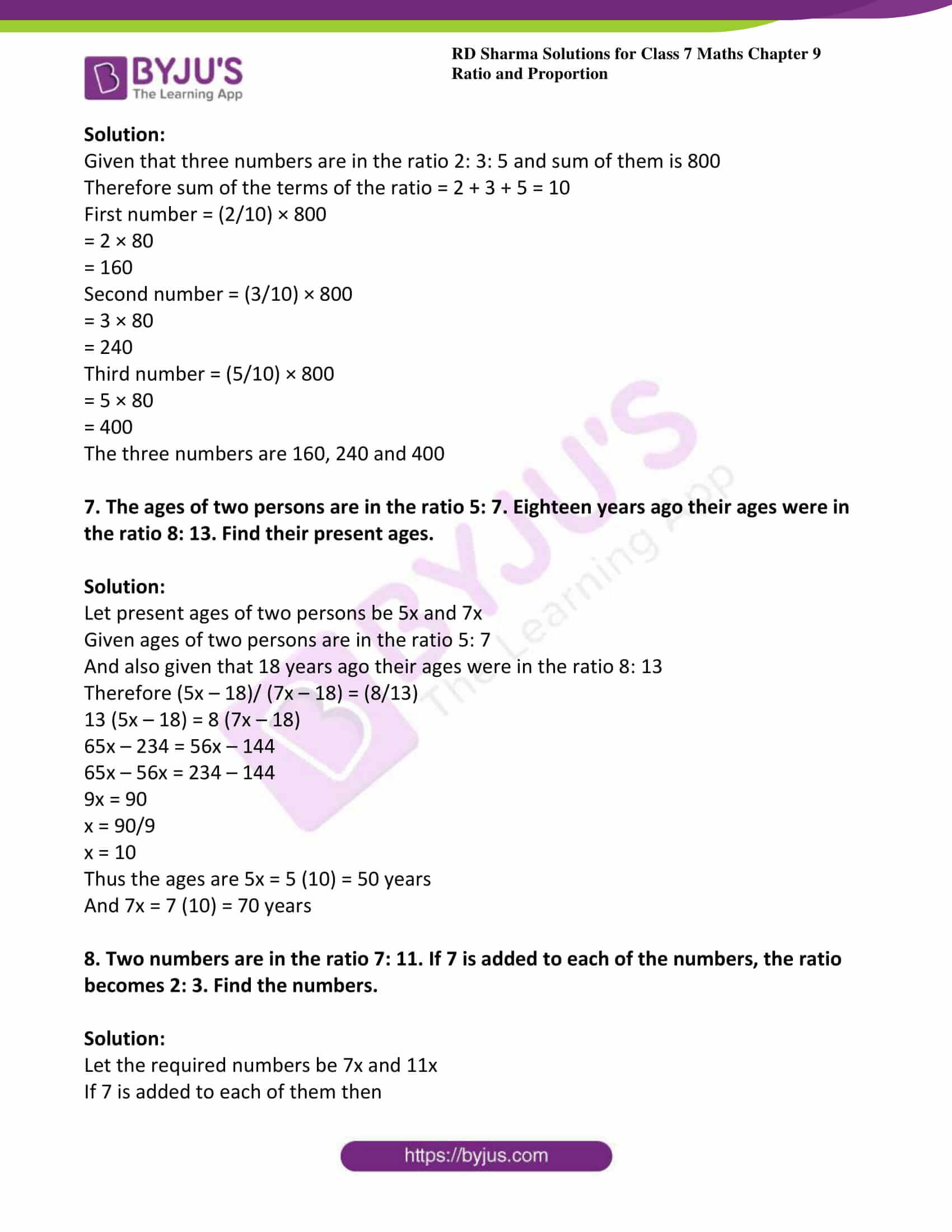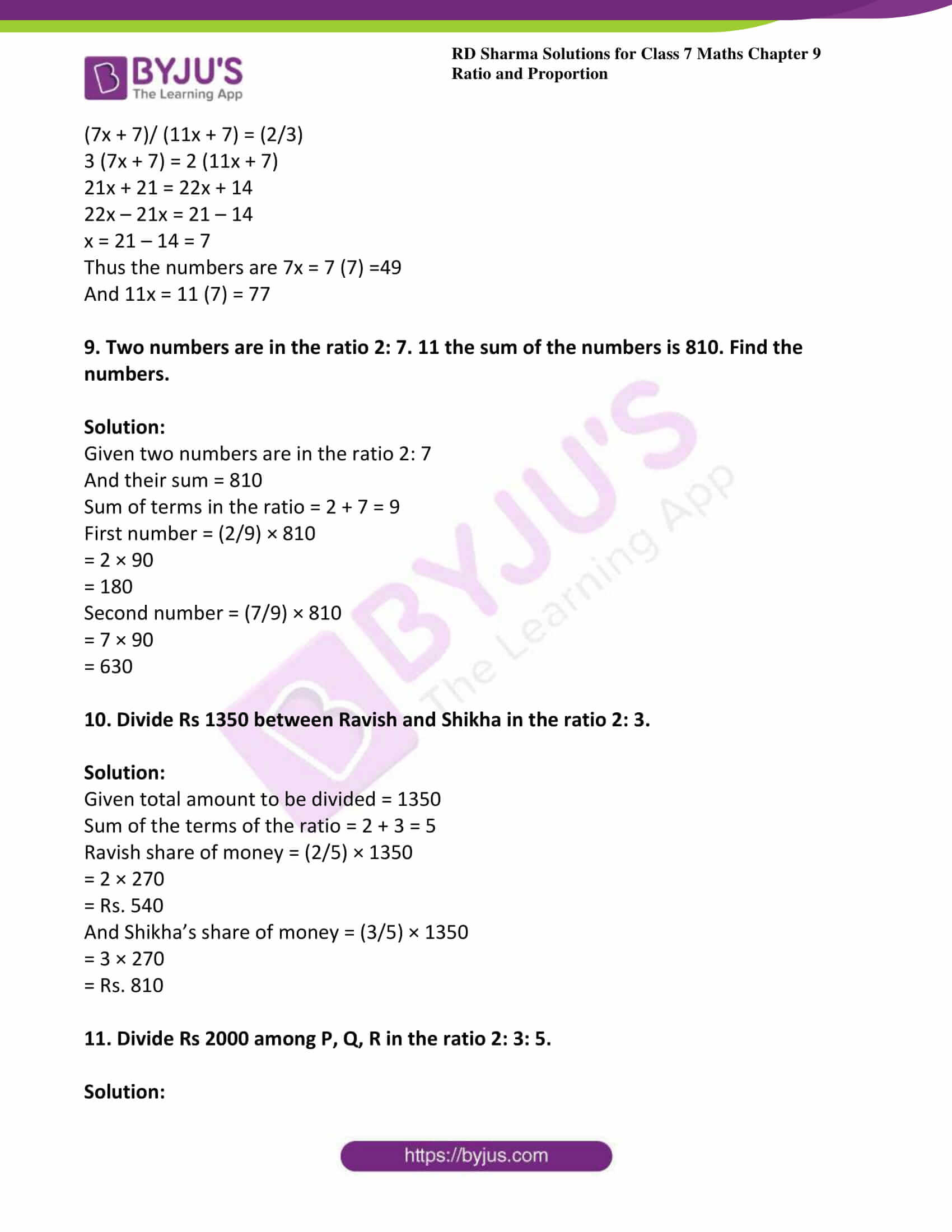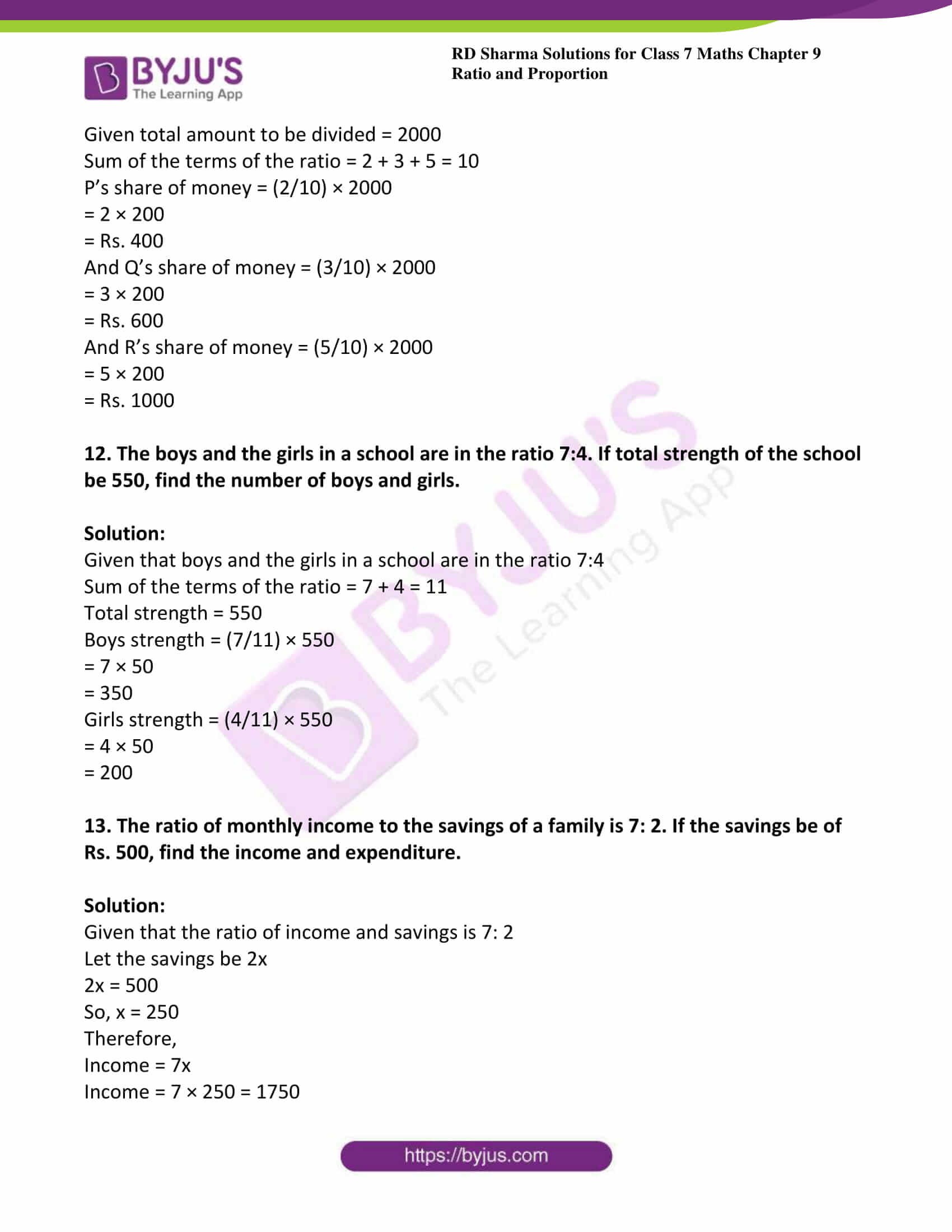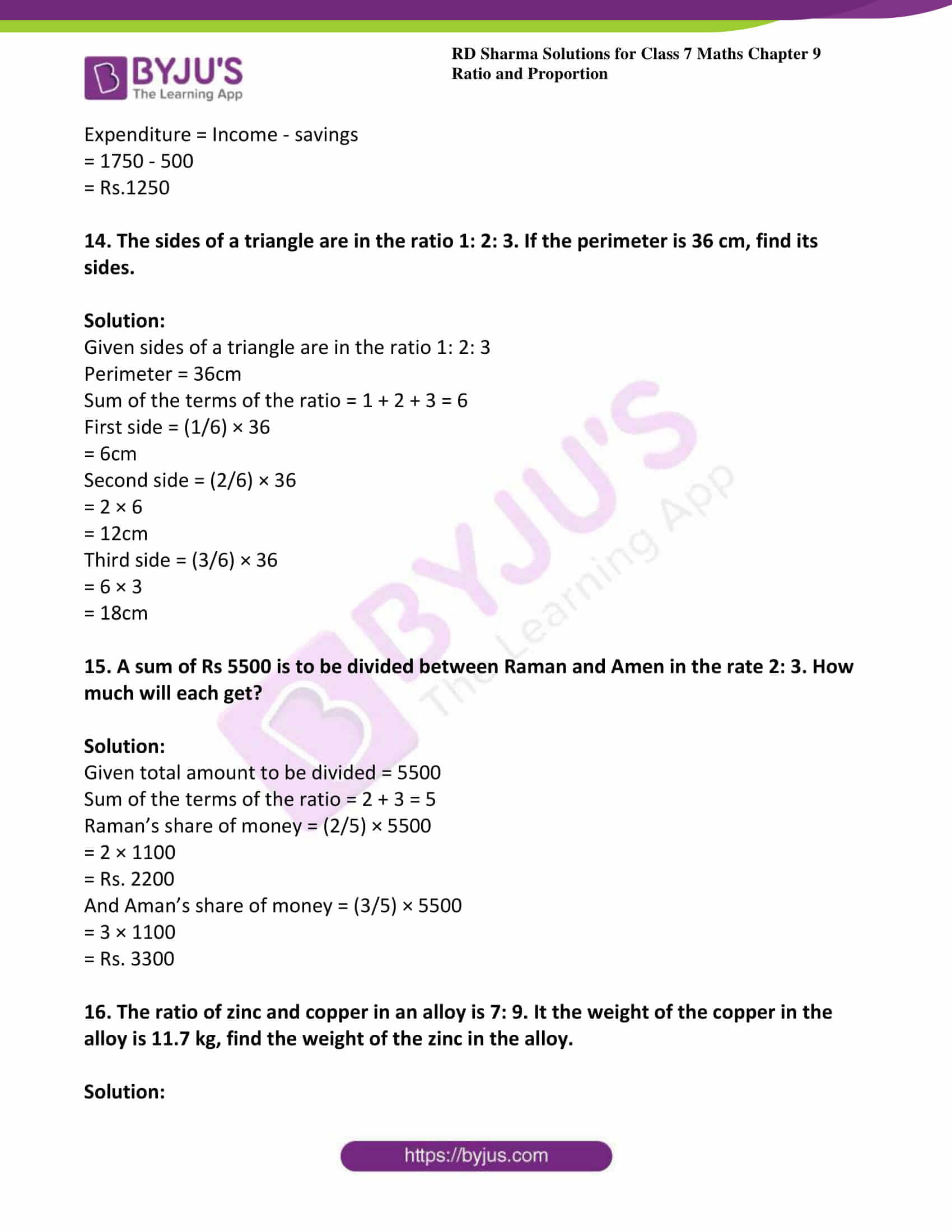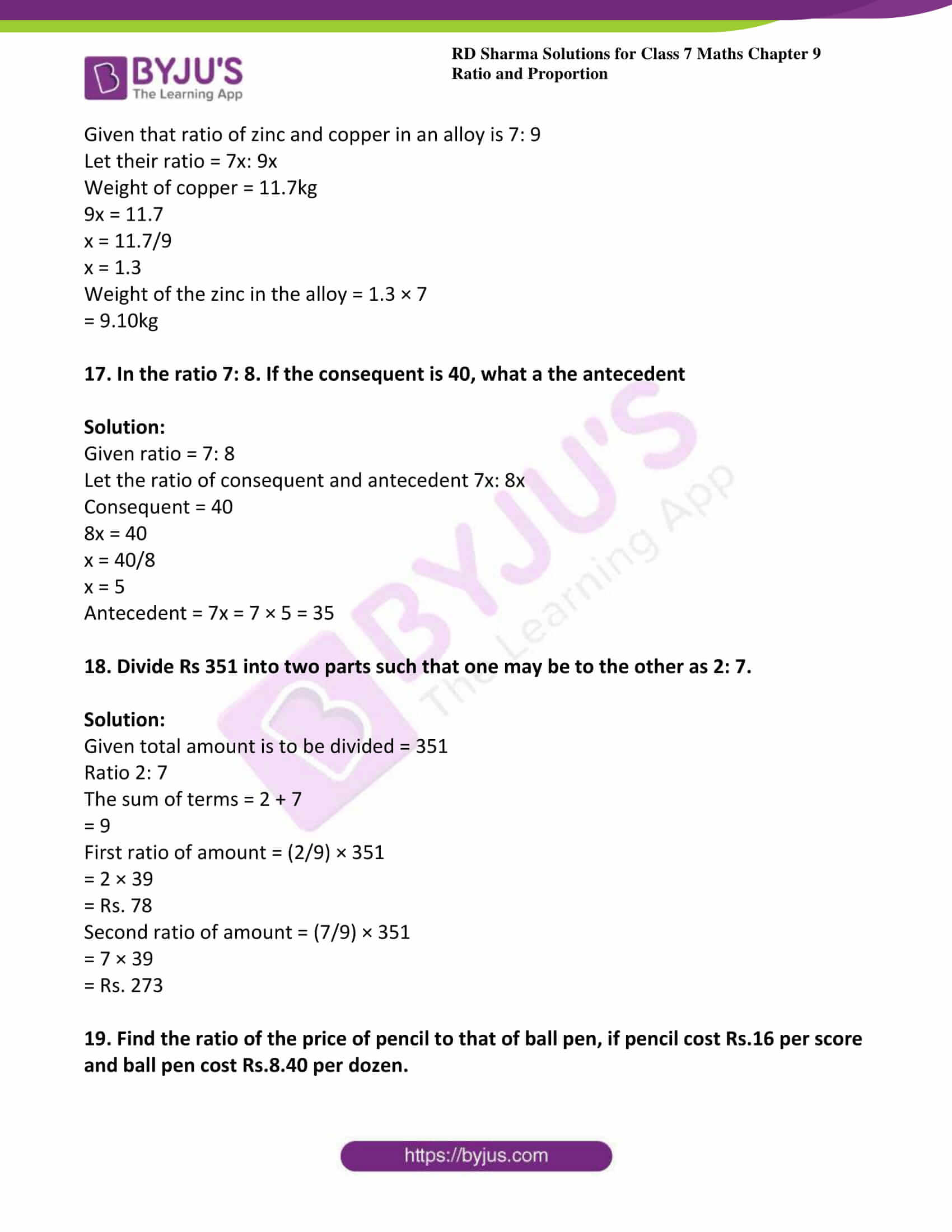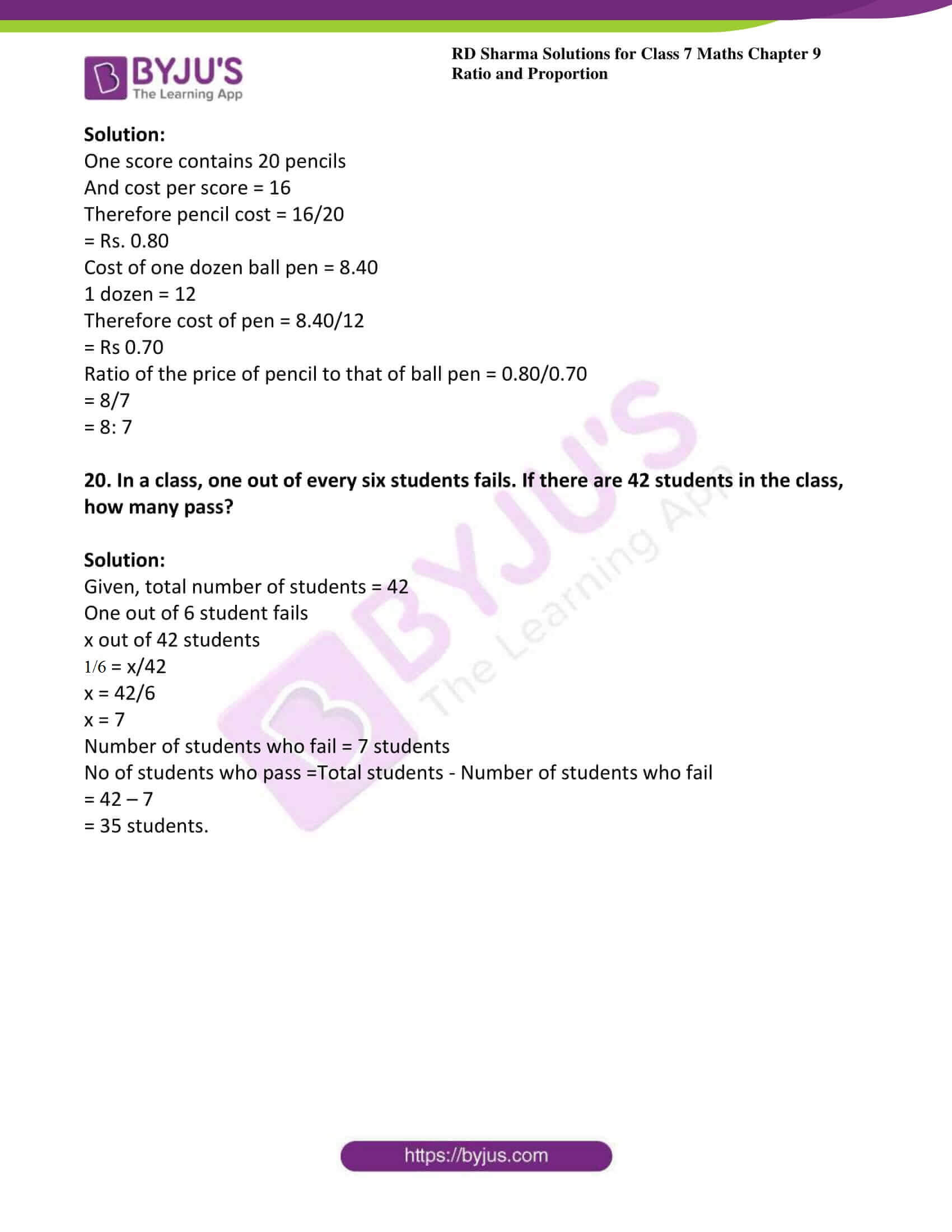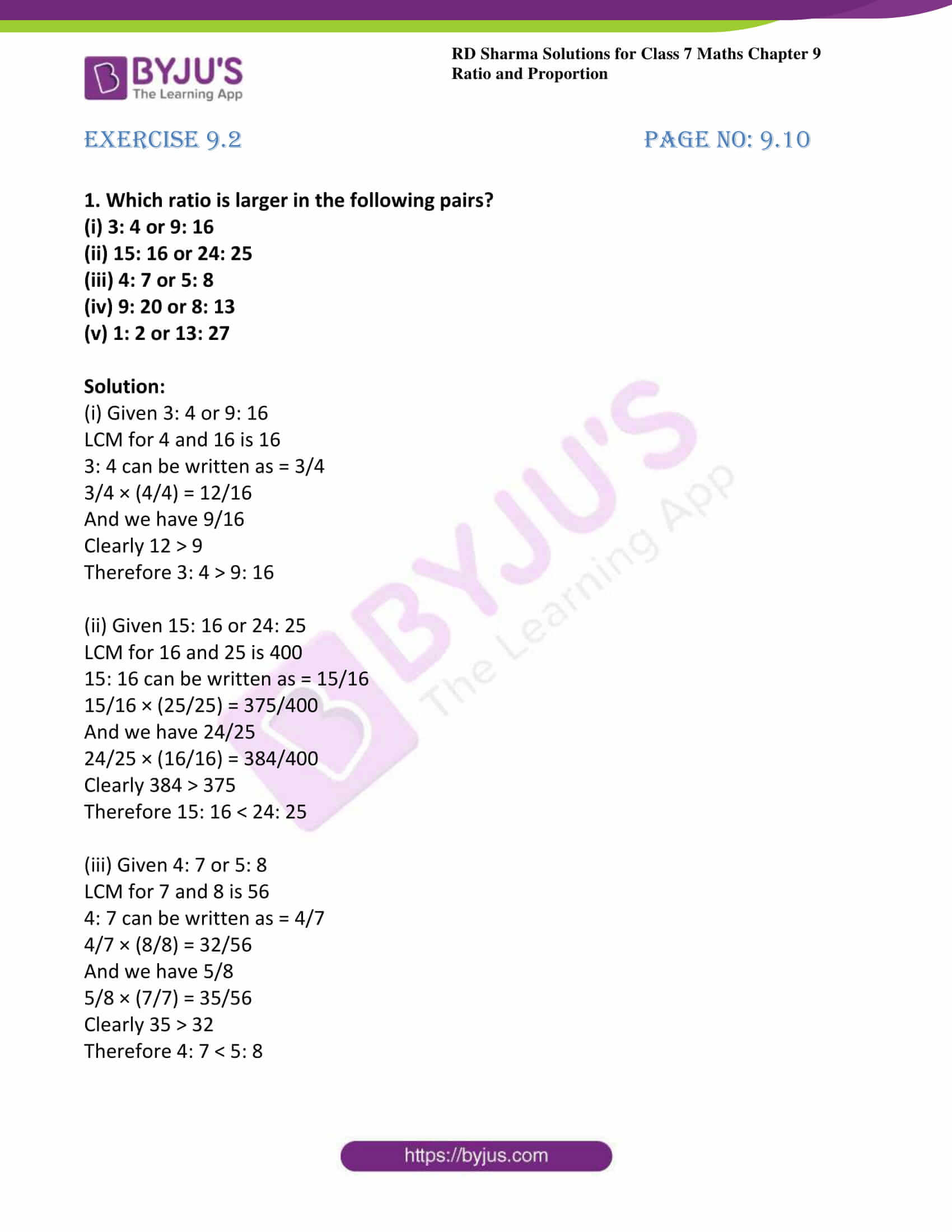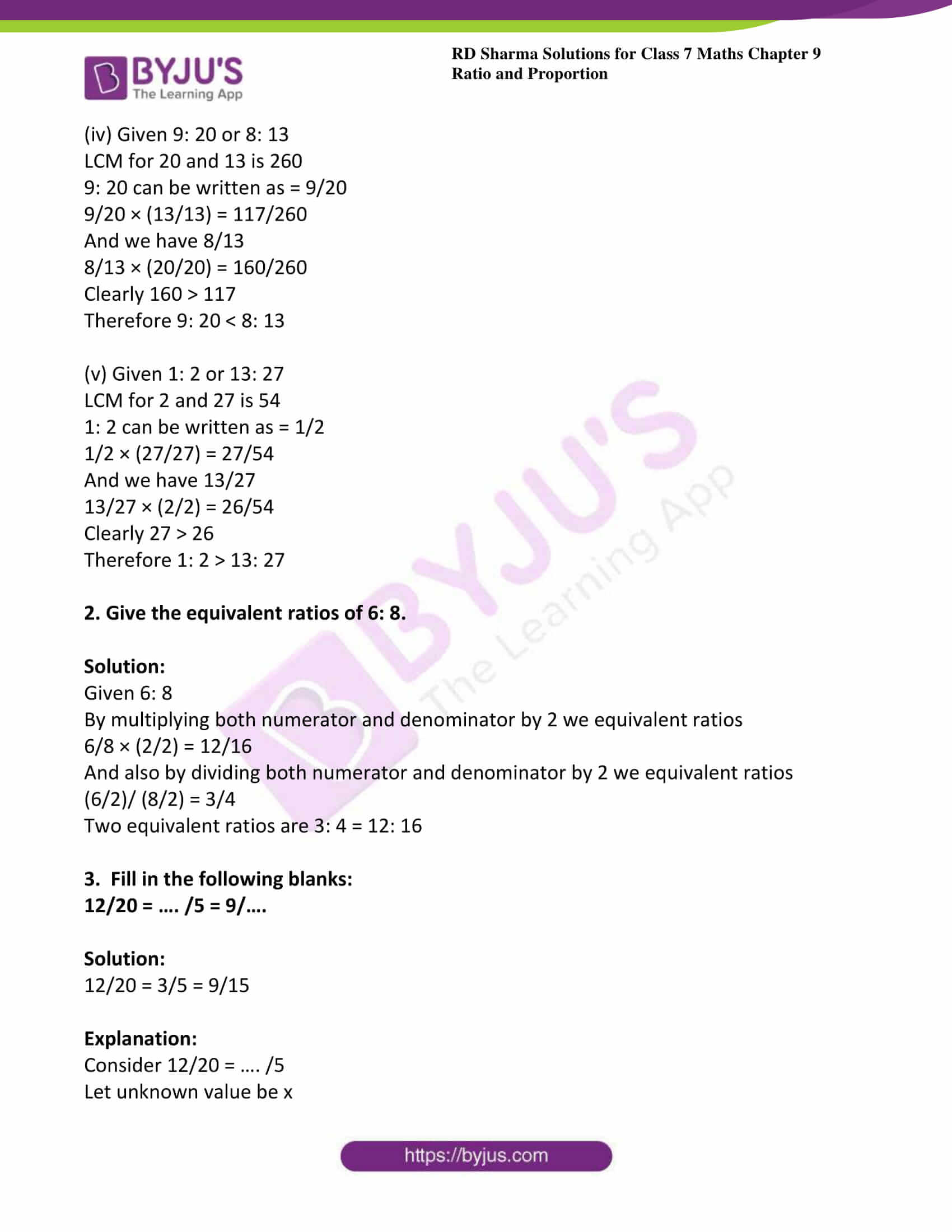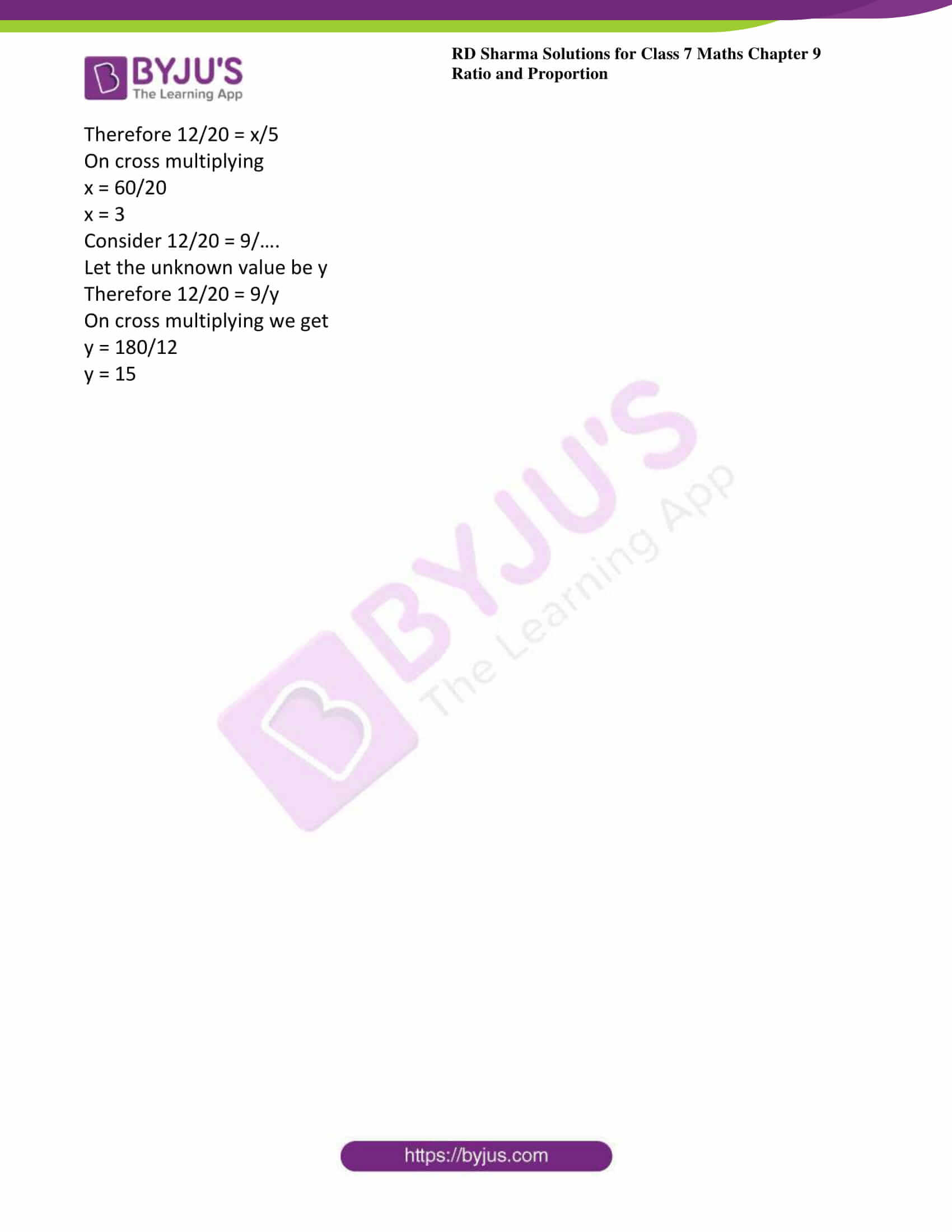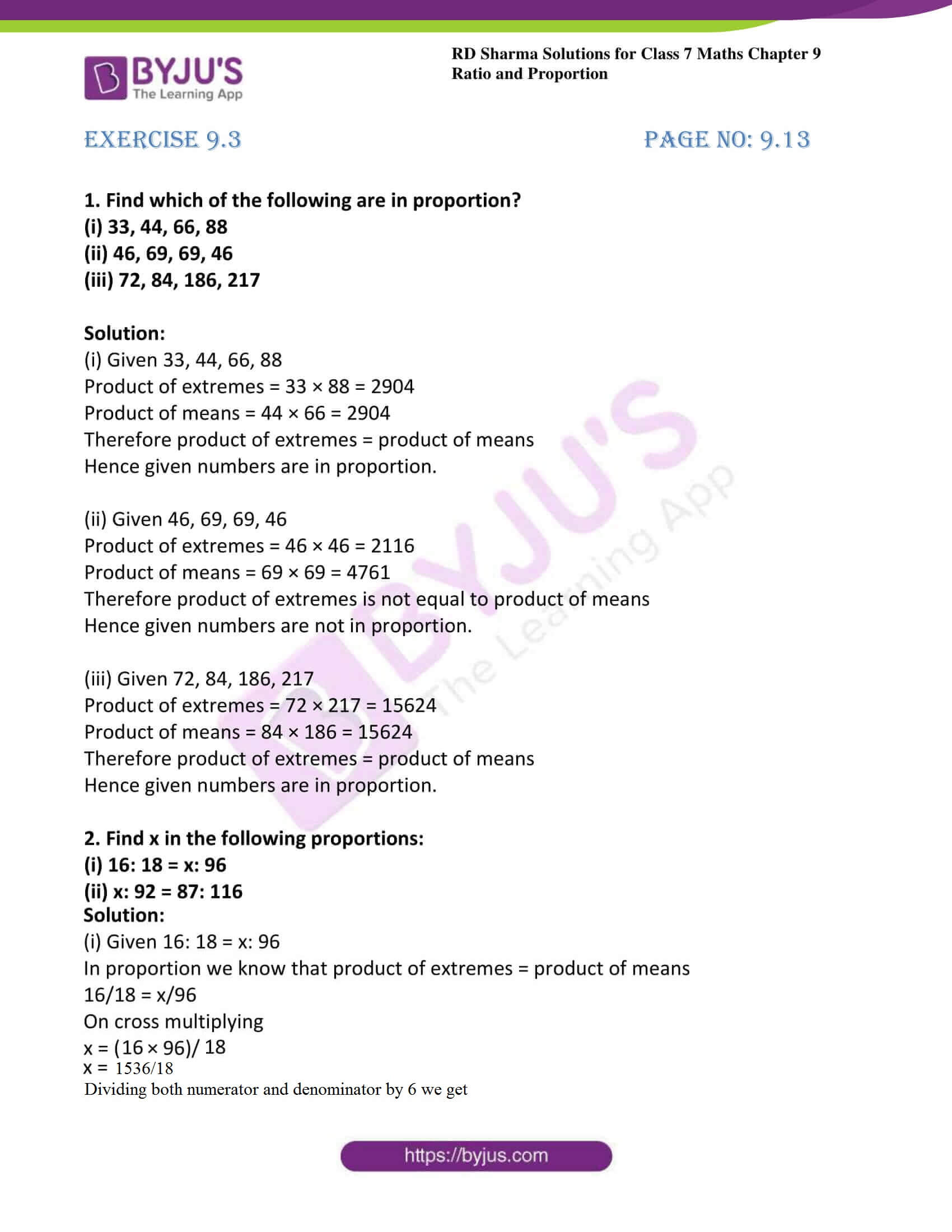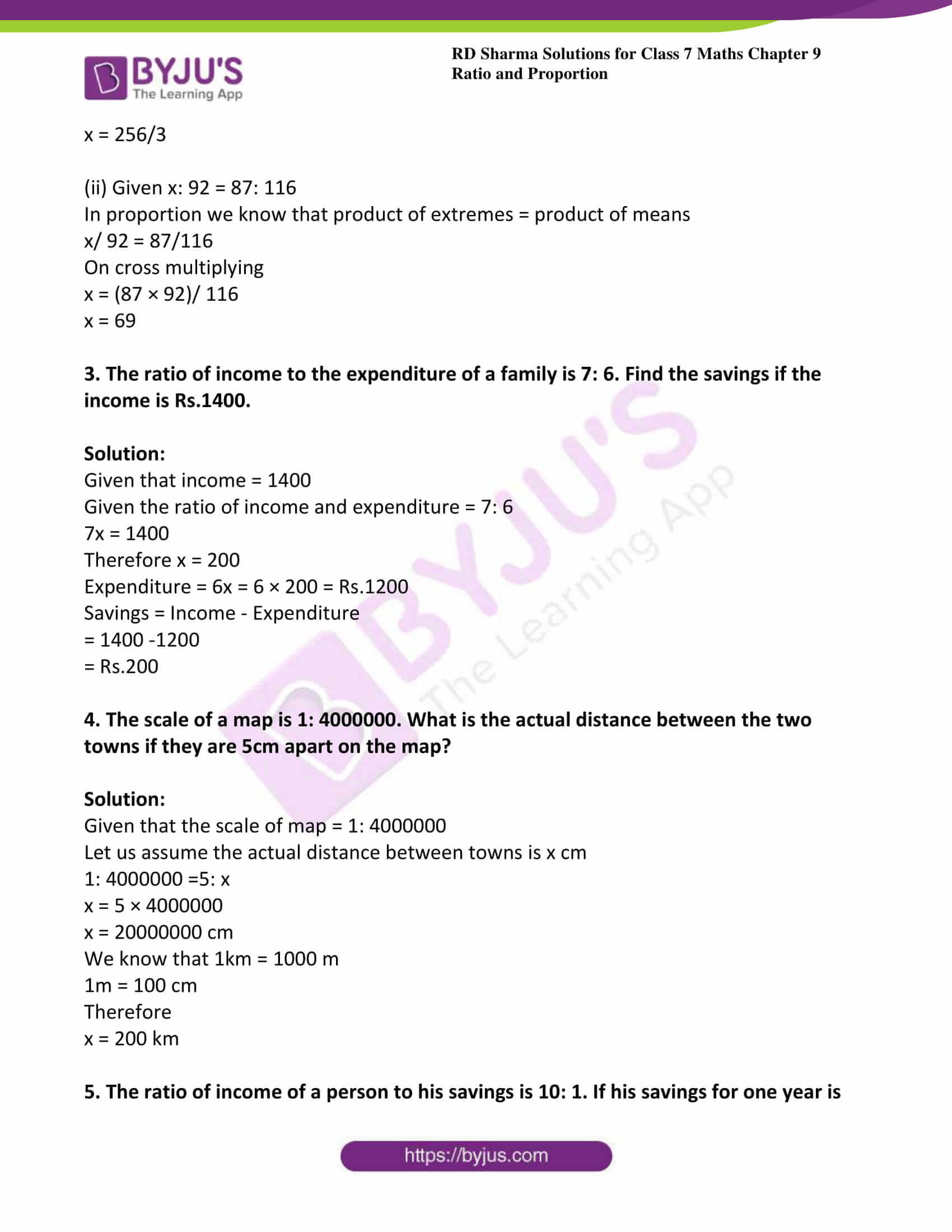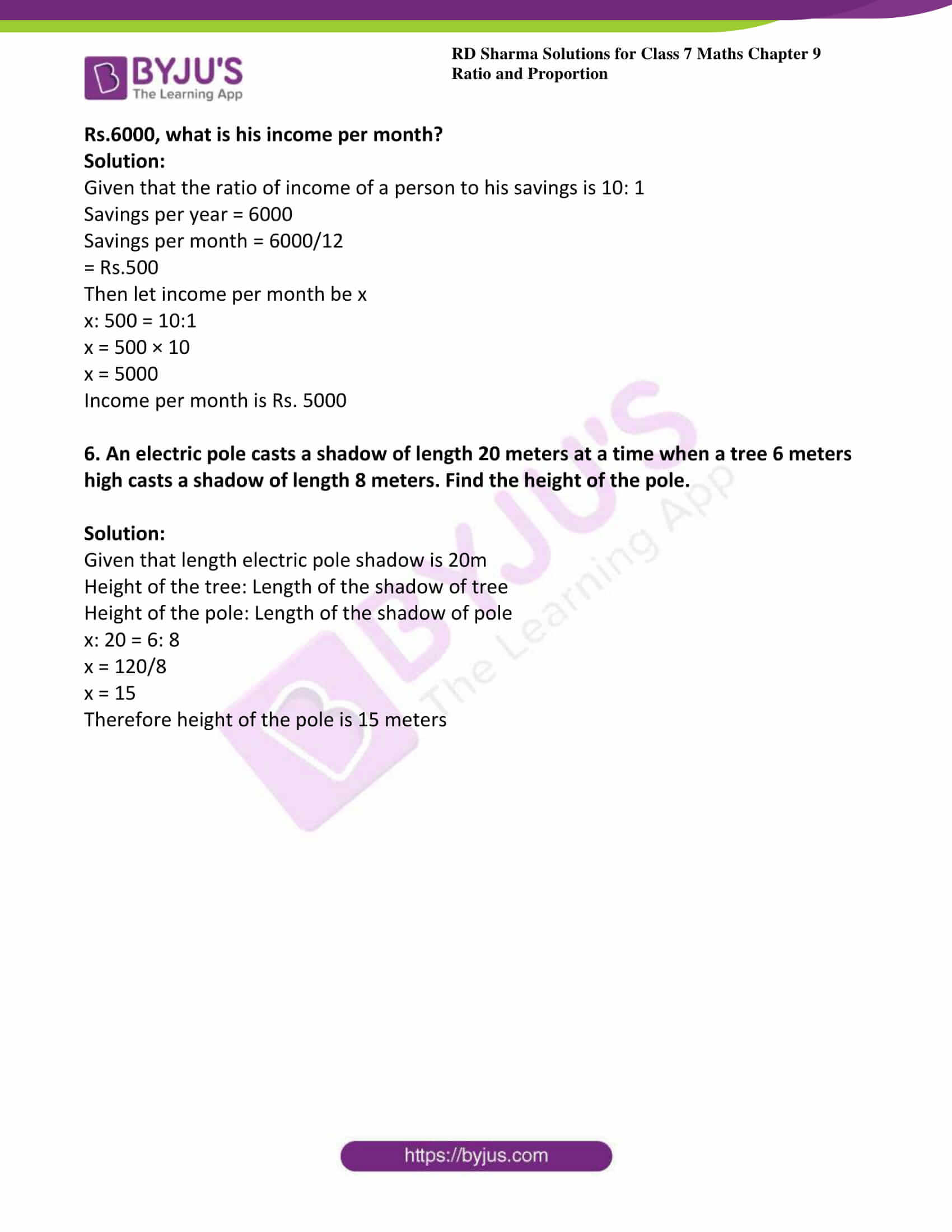### Access answers to Maths RD Sharma Solutions For Class 7 Chapter 9 – Ratio and Proportion

Exercise 9.1 Page No: 9.6

1. If x: y = 3: 5, find the ratio 3x + 4y: 8x + 5y

Solution:

Given x: y = 3: 5

We can write above equation as

x/y = 3/5

5x = 3y

x = 3y/5

By substituting the value of x in given equation 3x + 4y: 8x + 5y we get,

3x + 4y: 8x + 5y = 3 (3y/5) + 4y: 8 (3y/5) + 5y

= (9y + 20y)/5: (24y + 25y)/5

= 29y/5: 49y/5

= 29y: 49y

= 29: 49

2. If x: y = 8: 9, find the ratio (7x – 4y): 3x + 2y.

Solution:

Given x: y = 8: 9

We can write above equation as

x/y = 8/9

9x = 8y

x = 8y/9

By substituting the value of x in the given equation (7x – 4y): 3x + 2y we get,

(7x – 4y): 3x + 2y = 7 (8y/9) – 4y: 3 (8y/9) + 2y

= (56y – 36y)/9: 42y/9

= 20y/9: 42y/9

= 20y: 42y

= 20: 42

= 10: 21

3. If two numbers are in the ratio 6: 13 and their L.C.M is 312, find the numbers.

Solution:

Given two numbers are in the ratio 6: 13

Let the required number be 6x and 13x

The LCM of 6x and 13x is 78x

= 78x = 312

x = (312/78)

x = 4

Thus the numbers are 6x = 6 (4) = 24

13x = 13 (4) = 52

4. Two numbers are in the ratio 3: 5. If 8 is added to each number, the ratio becomes 2:3. Find the numbers.

Solution:

Let the required numbers be 3x and 5x

Given that if 8 is added to each other then ratio becomes 2: 3

That is 3x + 8: 5x + 8 = 2: 3

(3x + 8)/ (5x + 8) = 2/3

3 (3x + 8) = 2 (5x + 8)

9x + 24 = 10x + 16

By transposing

24 – 16 = 10x – 9x

x = 8

Thus the numbers are 3x = 3 (8) = 24

And 5x = 5 (8) = 40

5. What should be added to each term of the ratio 7: 13 so that the ratio becomes 2: 3

Solution:

Let the number to be added is x

Then (7 + x)/ (13 + x) = (2/3)

(7 + x) 3 = 2 (13 + x)

21 + 3x = 26 + 2x

3x – 2x = 26 – 21

x = 5

Hence the required number is 5

6. Three numbers are in the ratio 2: 3: 5 and the sum of these numbers is 800. Find the numbers

Solution:

Given that three numbers are in the ratio 2: 3: 5 and sum of them is 800

Therefore sum of the terms of the ratio = 2 + 3 + 5 = 10

First number = (2/10) × 800

= 2 × 80

= 160

Second number = (3/10) × 800

= 3 × 80

= 240

Third number = (5/10) × 800

= 5 × 80

= 400

The three numbers are 160, 240 and 400

7. The ages of two persons are in the ratio 5: 7. Eighteen years ago their ages were in the ratio 8: 13. Find their present ages.

Solution:

Let present ages of two persons be 5x and 7x

Given ages of two persons are in the ratio 5: 7

And also given that 18 years ago their ages were in the ratio 8: 13

Therefore (5x – 18)/ (7x – 18) = (8/13)

13 (5x – 18) = 8 (7x – 18)

65x – 234 = 56x – 144

65x – 56x = 234 – 144

9x = 90

x = 90/9

x = 10

Thus the ages are 5x = 5 (10) = 50 years

And 7x = 7 (10) = 70 years

8. Two numbers are in the ratio 7: 11. If 7 is added to each of the numbers, the ratio becomes 2: 3. Find the numbers.

Solution:

Let the required numbers be 7x and 11x

If 7 is added to each of them then

(7x + 7)/ (11x + 7) = (2/3)

3 (7x + 7) = 2 (11x + 7)

21x + 21 = 22x + 14

22x – 21x = 21 – 14

x = 21 – 14 = 7

Thus the numbers are 7x = 7 (7) =49

And 11x = 11 (7) = 77

9. Two numbers are in the ratio 2: 7. 11 the sum of the numbers is 810. Find the numbers.

Solution:

Given two numbers are in the ratio 2: 7

And their sum = 810

Sum of terms in the ratio = 2 + 7 = 9

First number = (2/9) × 810

= 2 × 90

= 180

Second number = (7/9) × 810

= 7 × 90

= 630

10. Divide Rs 1350 between Ravish and Shikha in the ratio 2: 3.

Solution:

Given total amount to be divided = 1350

Sum of the terms of the ratio = 2 + 3 = 5

Ravish share of money = (2/5) × 1350

= 2 × 270

= Rs. 540

And Shikha’s share of money = (3/5) × 1350

= 3 × 270

= Rs. 810

11. Divide Rs 2000 among P, Q, R in the ratio 2: 3: 5.

Solution:

Given total amount to be divided = 2000

Sum of the terms of the ratio = 2 + 3 + 5 = 10

P’s share of money = (2/10) × 2000

= 2 × 200

= Rs. 400

And Q’s share of money = (3/10) × 2000

= 3 × 200

= Rs. 600

And R’s share of money = (5/10) × 2000

= 5 × 200

= Rs. 1000

12. The boys and the girls in a school are in the ratio 7:4. If total strength of the school be 550, find the number of boys and girls.

Solution:

Given that boys and the girls in a school are in the ratio 7:4

Sum of the terms of the ratio = 7 + 4 = 11

Total strength = 550

Boys strength = (7/11) × 550

= 7 × 50

= 350

Girls strength = (4/11) × 550

= 4 × 50

= 200

13. The ratio of monthly income to the savings of a family is 7: 2. If the savings be of Rs. 500, find the income and expenditure.

Solution:

Given that the ratio of income and savings is 7: 2

Let the savings be 2x

2x = 500

So, x = 250

Therefore,

Income = 7x

Income = 7 × 250 = 1750

Expenditure = Income – savings

= 1750 – 500

= Rs.1250

14. The sides of a triangle are in the ratio 1: 2: 3. If the perimeter is 36 cm, find its sides.

Solution:

Given sides of a triangle are in the ratio 1: 2: 3

Perimeter = 36cm

Sum of the terms of the ratio = 1 + 2 + 3 = 6

First side = (1/6) × 36

= 6cm

Second side = (2/6) × 36

= 2 × 6

= 12cm

Third side = (3/6) × 36

= 6 × 3

= 18cm

15. A sum of Rs 5500 is to be divided between Raman and Amen in the rate 2: 3. How much will each get?

Solution:

Given total amount to be divided = 5500

Sum of the terms of the ratio = 2 + 3 = 5

Raman’s share of money = (2/5) × 5500

= 2 × 1100

= Rs. 2200

And Aman’s share of money = (3/5) × 5500

= 3 × 1100

= Rs. 3300

16. The ratio of zinc and copper in an alloy is 7: 9. It the weight of the copper in the alloy is 11.7 kg, find the weight of the zinc in the alloy.

Solution:

Given that ratio of zinc and copper in an alloy is 7: 9

Let their ratio = 7x: 9x

Weight of copper = 11.7kg

9x = 11.7

x = 11.7/9

x = 1.3

Weight of the zinc in the alloy = 1.3 × 7

= 9.10kg

17. In the ratio 7: 8. If the consequent is 40, what a the antecedent

Solution:

Given ratio = 7: 8

Let the ratio of consequent and antecedent 7x: 8x

Consequent = 40

8x = 40

x = 40/8

x = 5

Antecedent = 7x = 7 × 5 = 35

18. Divide Rs 351 into two parts such that one may be to the other as 2: 7.

Solution:

Given total amount is to be divided = 351

Ratio 2: 7

The sum of terms = 2 + 7

= 9

First ratio of amount = (2/9) × 351

= 2 × 39

= Rs. 78

Second ratio of amount = (7/9) × 351

= 7 × 39

= Rs. 273

19. Find the ratio of the price of pencil to that of ball pen, if pencil cost Rs.16 per score and ball pen cost Rs.8.40 per dozen.

Solution:

One score contains 20 pencils

And cost per score = 16

Therefore pencil cost = 16/20

= Rs. 0.80

Cost of one dozen ball pen = 8.40

1 dozen = 12

Therefore cost of pen = 8.40/12

= Rs 0.70

Ratio of the price of pencil to that of ball pen = 0.80/0.70

= 8/7

= 8: 7

20. In a class, one out of every six students fails. If there are 42 students in the class, how many pass?

Solution:

Given, total number of students = 42

One out of 6 student fails

x out of 42 students

1/6 = x/42

x = 42/6

x = 7

Number of students who fail = 7 students

No of students who pass =Total students – Number of students who fail

= 42 – 7

= 35 students.

Exercise 9.2 Page No: 9.10

1. Which ratio is larger in the following pairs?

(i) 3: 4 or 9: 16

(ii) 15: 16 or 24: 25

(iii) 4: 7 or 5: 8

(iv) 9: 20 or 8: 13

(v) 1: 2 or 13: 27

Solution:

(i) Given 3: 4 or 9: 16

LCM for 4 and 16 is 16

3: 4 can be written as = 3/4

3/4 × (4/4) = 12/16

And we have 9/16

Clearly 12 > 9

Therefore 3: 4 > 9: 16

(ii) Given 15: 16 or 24: 25

LCM for 16 and 25 is 400

15: 16 can be written as = 15/16

15/16 × (25/25) = 375/400

And we have 24/25

24/25 × (16/16) = 384/400

Clearly 384 > 375

Therefore 15: 16 < 24: 25

(iii) Given 4: 7 or 5: 8

LCM for 7 and 8 is 56

4: 7 can be written as = 4/7

4/7 × (8/8) = 32/56

And we have 5/8

5/8 × (7/7) = 35/56

Clearly 35 > 32

Therefore 4: 7 < 5: 8

(iv) Given 9: 20 or 8: 13

LCM for 20 and 13 is 260

9: 20 can be written as = 9/20

9/20 × (13/13) = 117/260

And we have 8/13

8/13 × (20/20) = 160/260

Clearly 160 > 117

Therefore 9: 20 < 8: 13

(v) Given 1: 2 or 13: 27

LCM for 2 and 27 is 54

1: 2 can be written as = 1/2

1/2 × (27/27) = 27/54

And we have 13/27

13/27 × (2/2) = 26/54

Clearly 27 > 26

Therefore 1: 2 > 13: 27

2. Give the equivalent ratios of 6: 8.

Solution:

Given 6: 8

By multiplying both numerator and denominator by 2 we equivalent ratios

6/8 × (2/2) = 12/16

And also by dividing both numerator and denominator by 2 we equivalent ratios

(6/2)/ (8/2) = 3/4

Two equivalent ratios are 3: 4 = 12: 16

3. Fill in the following blanks:

12/20 = …. /5 = 9/….

Solution:

12/20 = 3/5 = 9/15

Explanation:

Consider 12/20 = …. /5

Let unknown value be x

Therefore 12/20 = x/5

On cross multiplying

x = 60/20

x = 3

Consider 12/20 = 9/….

Let the unknown value be y

Therefore 12/20 = 9/y

On cross multiplying we get

y = 180/12

y = 15

Exercise 9.3 Page No: 9.13

1. Find which of the following are in proportion?

(i) 33, 44, 66, 88

(ii) 46, 69, 69, 46

(iii) 72, 84, 186, 217

Solution:

(i) Given 33, 44, 66, 88

Product of extremes = 33 × 88 = 2904

Product of means = 44 × 66 = 2904

Therefore product of extremes = product of means

Hence given numbers are in proportion.

(ii) Given 46, 69, 69, 46

Product of extremes = 46 × 46 = 2116

Product of means = 69 × 69 = 4761

Therefore product of extremes is not equal to product of means

Hence given numbers are not in proportion.

(iii) Given 72, 84, 186, 217

Product of extremes = 72 × 217 = 15624

Product of means = 84 × 186 = 15624

Therefore product of extremes = product of means

Hence given numbers are in proportion.

2. Find x in the following proportions:

(i) 16: 18 = x: 96

(ii) x: 92 = 87: 116

Solution:

(i) Given 16: 18 = x: 96

In proportion we know that product of extremes = product of means

16/18 = x/96

On cross multiplying

x = (16 × 96)/ 18

x = 1536/18

Dividing both numerator and denominator by 6

x = 256/3

(ii) Given x: 92 = 87: 116

In proportion we know that product of extremes = product of means

x/ 92 = 87/116

On cross multiplying

x = (87 × 92)/ 116

x = 69

3. The ratio of income to the expenditure of a family is 7: 6. Find the savings if the income is Rs.1400.

Solution:

Given that income = 1400

Given the ratio of income and expenditure = 7: 6

7x = 1400

Therefore x = 200

Expenditure = 6x = 6 × 200 = Rs.1200

Savings = Income – Expenditure

= 1400 -1200

= Rs.200

4. The scale of a map is 1: 4000000. What is the actual distance between the two towns if they are 5cm apart on the map?

Solution:

Given that the scale of map = 1: 4000000

Let us assume the actual distance between towns is x cm

1: 4000000 =5: x

x = 5 × 4000000

x = 20000000 cm

We know that 1km = 1000 m

1m = 100 cm

Therefore

x = 200 km

5. The ratio of income of a person to his savings is 10: 1. If his savings for one year is Rs.6000, what is his income per month?

Solution:

Given that the ratio of income of a person to his savings is 10: 1

Savings per year = 6000

Savings per month = 6000/12

= Rs.500

Then let income per month be x

x: 500 = 10:1

x = 500 × 10

x = 5000

Income per month is Rs. 5000

6. An electric pole casts a shadow of length 20 meters at a time when a tree 6 meters high casts a shadow of length 8 meters. Find the height of the pole.

Solution:

Given that length electric pole shadow is 20m

Height of the tree: Length of the shadow of tree

Height of the pole: Length of the shadow of pole

x: 20 = 6: 8

x = 120/8

x = 15

Therefore height of the pole is 15 meters